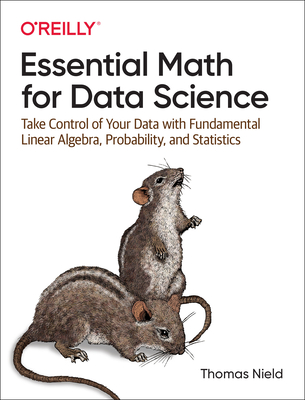### Essential Math for Data Science: Take Control of Your Data with Fundamental Linear Algebra, Probability, and Statistics### Huge savings for students

Each student receives a 50% discount off of most books in the HSG Book Store. During class, please ask the instructor about purchase details.
 List Price: \$59.99 Price: \$30.00 You Save: \$30.00
3

Master the math needed to excel in data science, machine learning, and statistics. In this book author Thomas Nield guides you through areas like calculus, probability, linear algebra, and statistics and how they apply to techniques like linear regression, logistic regression, and neural networks. Along the way you'll also gain practical insights into the state of data science and how to use those insights to maximize your career.

Learn how to:

• Use Python code and libraries like SymPy, NumPy, and scikit-learn to explore essential mathematical concepts like calculus, linear algebra, statistics, and machine learning
• Understand techniques like linear regression, logistic regression, and neural networks in plain English, with minimal mathematical notation and jargon
• Perform descriptive statistics and hypothesis testing on a dataset to interpret p-values and statistical significance
• Manipulate vectors and matrices and perform matrix decomposition
• Integrate and build upon incremental knowledge of calculus, probability, statistics, and linear algebra, and apply it to regression models including neural networks
• Navigate practically through a data science career and avoid common pitfalls, assumptions, and biases while tuning your skill set to stand out in the job market
O'Reilly Media